#### Adding vectors using trigonometric methods

A) Pythagoras's Theorem & TrigonometryReview: Mathematical concepts in trigonometry

Recall Pythagoras's Theorem: C2 = A2  + B

This theorem applies only to right angle triangles like the one below.
In other words, it can be used only when vector A is perpendicular to vector BThe three basic trigonometric identities that can be derived from a right angle triangle whose included angle is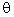, are: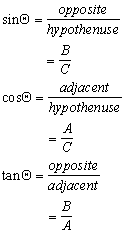B) Sine and Cosine Laws MethodFor triangles that are NOT right-angle triangles we can use modified trigonometric laws known as the cosine and sine laws:

The triangle below is a generalized isosceles triangle where none of the sides are perpendicular to one another.Note that we use lower case letters to represent the sides of the triangle and upper case letters to represent the included angles.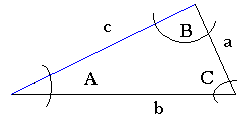Memorize these two laws ... they will come in very handy

1. The sine Law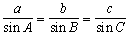2. The Cosine Law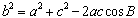Example: What was the total displacement of a balloon that drifts for 7 km in a [NE] direction and then is pushed by a wind in a southerly direction for another 3 km.In case you forgot: [NE] is a direction half way between the y axis [N] and the x axis  [E] ---> [N450E]Use the sine/cosine laws to solve this problem.

Solution:Knowing the rules of angles here is also important.  For example the Opposite Angles Theorem tells us that the angle between d1 and d2 (angle B) is also  450 - see diagram below: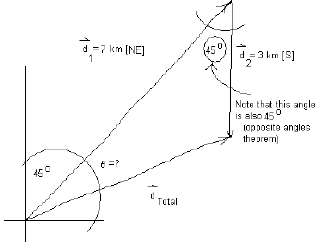Step-by-step ...

1. ##### We first apply  the cosine Law to find the magnitude of the resultant vector, dtotal

where:

b = dtotal (to be calculated)

a = 3 km [S];

c = 7 km [NE]
and

B = 450

therefore applying the cosine law

#### b2 = (3)2 + ( 7)2 - 2(3)(7)(cos(450))

and b2 = 28.3

and [ by taking the square root of b2]

b = 5.32 km   ----> this is the magnitude of dtotal

##### 2. Finding the direction of the resultant - Apply the Sine Law to find the value of the anglefrom the general equationwe can only use two of the terms for which we have information: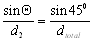cross multiply, isolate,  and solve for sinsin= 0.398

Now use the inverse of sin (sin-1) to find the angle in degrees

#####= invsin(0.398) = 23.500

##### 3. Make a final statement indicating your findingsthe vector  dtotal has direction (450 + 23.50) --- see diagram below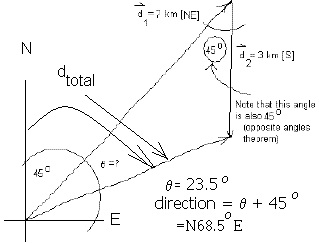dtotal = 5.32 km [N68.50]